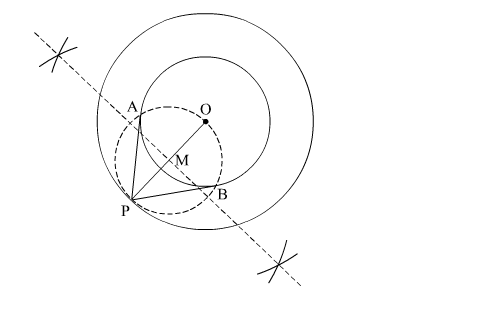# Draw two concentric circles of radii 3 cm and 5 cm.`
Question:

Draw two concentric circles of radii 3 cm and 5 cm. Taking a point on the outer circle, construct the pair of tangents to the inner circle

Solution:

Steps of Construction

Step 1. Mark a point O on paper.

Step 2. Taking O as a centre, draw two concentric circles of radius 3 cm and 5 cm. Mark any random point P on outer circle.

Step 3. Join OP and draw its perpendicular bisector which meets OP at M.

Step 4. Draw a circle with M as centre and radius PM (or OM), to intersect the inner circle at the points A and B.

Step 5. Join PA and PB.Here, PA and PB are the required tangents.